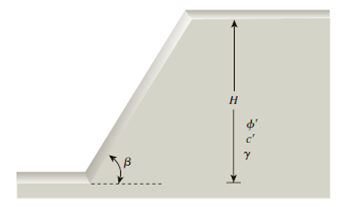Chapter 15, Problem 15.10PPrinciples of Geotechnical Enginee...

9th Edition
Braja M. Das + 1 other
ISBN: 9781305970939

Solutions

Chapter
SectionPrinciples of Geotechnical Enginee...

9th Edition
Braja M. Das + 1 other
ISBN: 9781305970939
Textbook Problem

For the finite slope shown in Figure 15.50, assume that the slope failure would occur along a plane (Culmann’s assumption). Find the height of the slope for critical equilibrium. Given: β = 49°, γ = 17.6 kN/m3, ϕ ′ = 19 ° , and c′ = 42 kN/m2.Figure 15.50

To determine

Find the height Hcr of the slope for critical equilibrium.

Explanation

Given information:

The slope with an inclination β is 49°.

The angle of friction ϕ is 19°.

The cohesion c is 42kN/m2.

The unit weight γ of the soil is 17.6kN/m3.

Calculation:

Determine the height Hcr of the slope for critical equilibrium using the formula.

Hcr=4cγ[sinβcosϕ1cos(βϕ)]

Substitute 42kN/m2 for c, 17

Still sussing out bartleby?

Check out a sample textbook solution.

See a sample solution

The Solution to Your Study Problems

Bartleby provides explanations to thousands of textbook problems written by our experts, many with advanced degrees!

Get Started

What are the most popular encryption systems used over the Web?

Principles of Information Security (MindTap Course List)

Describe how game theory might be used in a business setting.

Principles of Information Systems (MindTap Course List)

What are two reasons for using an air spring?

Automotive Technology: A Systems Approach (MindTap Course List)

What is structural independence, and why is it important?

Database Systems: Design, Implementation, & Management

What is an RFP, and how does it differ from an RFQ?

Systems Analysis and Design (Shelly Cashman Series) (MindTap Course List)

What techniques can you use to improve search results? (72)

Enhanced Discovering Computers 2017 (Shelly Cashman Series) (MindTap Course List)

What emergency steps should be taken to treat burns?

Welding: Principles and Applications (MindTap Course List)